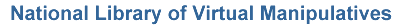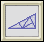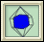﻿ NLVM 9 - 12 - Geometry ManipulativesCob Web Plot – Change variables and observe patterns from this graphing simulation.Fractals - Iterative – Generate six different fractals.Fractals - Koch and Sierpinski – Change colors and pause this fractal simulation at any point.Fractals - Mandelbrot and Julia Sets – Investigate relationships between these two fractal sets.Fractals - Polygonal – Change the parameters to create a new fractal.Geoboard – Use geoboards to illustrate area, perimeter, and rational number concepts.Geoboard - Circular – Use circular geoboards to illustrate angles and degrees.Geoboard - Coordinate – Rectangular geoboard with x and y coordinates.Geoboard - Isometric – Use geoboard to illustrate three-dimensional shapes.Golden Rectangle – Illustrates iterations of the Golden Section.Great Circle – Use a 3D globe to visualize and measure the shortest path between cities.Pattern Blocks – Use six common geometric shapes to build patterns and solve problems.Pinwheel Tiling – Construct and explore a very unusual tiling of the plane by right triangles.Platonic Solids – Identify characteristics of the Platonic Solids.Platonic Solids - Duals – Identify the duals of the platonic solids.Platonic Solids - Slicing – Discover shapes and relationships between slices of the platonic solids.Polyominoes – Build and compare characteristics of biominoes, triominoes, quadrominoes, etc.Pythagorean Theorem – Solve two puzzles that illustrate the proof of the Pythagorean Theorem.Right Triangle Solver – Practice using the Pythagorean theorem and the definitions of the trigonometric functions to solve for unknown sides and angles of a right triangle.Space Blocks – Create and discover patterns using three dimensional blocks.Tangrams – Use all seven Chinese puzzle pieces to make shapes and solve problems.Tessellations – Using regular and semi-regular tessellations to tile the plane.Tight Weave – Visualize the creation of the Sierpinski Carpet, an iterative geometric pattern that resembles a woven mat.Transformations - Composition – Explore the effect of applying a composition of translation, rotation, and reflection transformations to objects.Transformations - Dilation – Dynamically interact with and see the result of a dilation transformation.Transformations - Reflection – Dynamically interact with and see the result of a reflection transformation.Transformations - Rotation – Dynamically interact with and see the result of a rotation transformation.Transformations - Translation – Dynamically interact with and see the result of a translation transformation.Triangle Solver – Practice using the law of sines and the law of cosines to solve for unknown sides and angles of a triangle.Turtle Geometry – Explore numbers, shapes, and logic by programming a turtle to move.